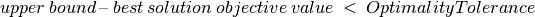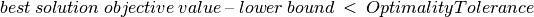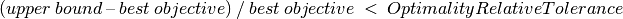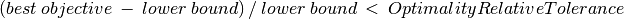# Optimizing with Artelys Kalis¶

In this section, you will find all relevant information to perform optimization with Artelys Kalis. This includes:

• how to define an objective function and associate it to a problem ;

• how to start the optimization process ;

• how to limit the tree search algorithm in order to obtain acceptable computation times;

• how to specify tolerances for the optimality of the solution ;

• how to get statistics about the solving process.

All features are going to be illustrated by a simple scheduling example.

## A scheduling problem with time windows¶

This problem will be used as example throughout this chapter. We will briefly describe how to build the problem using classes which have been described in the previous chapter and then explore in depth the optimization techniques available in Artelys Kalis.

Let us assume that you are in charge of a project composed of seven tasks, each of them being mandatory. Each task has a duration, a release date and a due date. The objective is to carry this project through to a successful conclusion as soon as possible, by deciding the start date of each task: the start date of each task is a decision variable of the problem.

Here are the constraints that must be taken into account:

• Each task must begin after its release date.

• Each task must be finished before its due date.

• The last task is the delivery date of the project: all six other tasks must be finished before the last one begins.

• Some tasks cannot begin before another task has ended. These are: task 1 and 2 cannot be started before task 0 has finished, task 4 must be started after the end of task 2, task 5 cannot start before task 1 has ended and finally task 3 can take place only after the end of task 4. We call these constraints precedence constraints.

• One can do only one task at a time: for each couple of tasks which could be performed at the same time considering the constraints which we have already stated, we add a disjunction constraint forbidding it. These couples are tasks 1 and 2, 4 and 5, 1 and 4, 2 and 5, 3 and 5, 1 and 3.

“Carrying the project to successful conclusion as soon as possible” means ending the last task as early as possible. This is equivalent to start it as early as possible. So not only do we have to find a start date for each task in such a way that all constraints are satisfied (solve the problem), but we must choose one solution, among all of them, which minimizes the start date of the last task. This is what we call optimizing the problem, and the start date of the last task is the objective which we try to minimize.

In the following sections, we show how Artelys Kalis can be used to perform such optimizations within a C++, Python or Java program.

## Building the problem¶

If you have not read Chapter 3, “Solving a constraint problem”, we recommend that you do so before carrying on with this chapter. Below we use concepts and classes which have been explained in Chapter 3.

Here are some variables we declare at the beginning of our program to make it more concise in the reminder.

```int nbTasks = 7;
int releaseDates = {0,4,0,6,3,7,0};
int dueDates = {4,9,3,12,12,20,30};
int durations = {2,2,1,4,3,5,0};
```
```int nbPrecedence = 5;
int precedencesFirstElements = {0,0,2,1,4};
int precedencesSecondElements = {1,2,4,5,3};
```
```int nbDisjunctions = 6;
int disjunctionsFirstElements = {1,5,1,2,5,1};
int disjunctionsSecondElements = {2,4,4,5,3,3};
```

For the tasks, we have declared:

• their number: `nbTasks` ;

• their release dates: `releaseDates` ;

• their due dates: `dueDates` ;

• their durations: `durations`.

For the precedence constraints, we have declared:

• their number: `nbPrecedence` ;

• the tasks to be performed first for each constraint: `precedencesFirstElements` ;

• the tasks to be performed second for each constraint: `precedencesSecondElements`.

Therefore, for the ith precedence constraint, the task `precedencesFirstElements[i]` must be performed before the task `precedencesSecondElements[i]`. Note that the tasks are numbered from 0 to 6.

For the disjunction constraints, we have declared:

• their number: `nbDisjunctions` ;

• the tasks that cannot be performed at the same time: `disjunctionsFirstElements` and `disjunctionsSecondElements`.

The ith disjunction constraint will state that the tasks `disjunctionsFirstElements[i]` and `disjunctionsSecondElements[i]` cannot be performed at the same time.

Now we can construct the Artelys Kalis objects to state our scheduling problem:

```KProblem scheduling(session, "Scheduling");
KIntVarArray startDates(scheduling,7,0,100,"StartDate");
```

Here we have built our `KProblem` object `scheduling` and the `KIntVarArray` `startDates` that contains the seven decision variables representing the start dates of each task. These start dates can be between 0 and 100. We can then add the constraints to our problem. These are the constraints stating that “each task must begin after its release date” and that “each task must end before its due date”:

```for(taskIndex=0; taskIndex < nbTasks; taskIndex++)
{
}
```

These are the constraints stating that “each of the six first tasks must end before the last task may start”:

```for(taskIndex=0; taskIndex < nbTasks-1; taskIndex++)
{
}
```

These are the precedence constraints:

```for(taskIndex=0; taskIndex < nbPrecedence; taskIndex++)
{
}
```

Finally, these are the disjunction constraints:

```for(taskIndex=0; taskIndex < nbDisjunctions; taskIndex++)
{
}
```

The objective of an optimization problem is a decision variable that we try to minimize (or to maximize). Here our objective is simply made up of the start date of the last task (`startDates`).

We can specify the objective variable by calling the `setObjective` method:

```scheduling.setObjective(startDates);
```

We wish to minimize this objective. The minimization is the default sense for the optimization, but we could also specify it by using the `setSense` method:

```scheduling.setSense(KProblem::Minimize);
```

Another example: if we wish to maximize the time interval between the start date of task 0 and the start date of task 3, we would have written:

```KIntVar varObj;
scheduling.post(varObj == startDates-startDates);
scheduling.setObjective(varObj);
scheduling.setSense(KProblem::Maximize);
```

## Optimality tolerance¶

Sometimes it is not worth searching for the very best solution (exact optimization). If retrieving a good solution (approximate optimization) meets your needs it is very important to store this information in the `KSolver` object. This may leads to avoid the exploration of a great number of nodes thereby reducing significantly computation time.

Two control parameters specify what is considered to be a good solution. Both are based on the fact that, at each node of the search tree, the solver knows an upper and a lower bound of the objective value. In maximization (respectively in minimization) the quality of the current best solution is as good as the objective value is close to the upper (respectively lower) bound. The two control parameters set a limit on this quality.

The first control is `KSolver::OptimalityTolerance` and is a double control. If this value is set, the optimization algorithm will not expand nodes for which:and the minimization algorithm will not explore nodes for which:This tolerance is called an absolute tolerance. In our example, setting this control to 2 would state that “solutions in which the project ends two time units (hours) later than the minimum time are good solutions”.

The second control is `KSolver::OptimalityRelativeTolerance` and is a double control. If this value is set, the maximization algorithm will not expand nodes for which:and the minimization algorithm will not explore nodes for which:This tolerance is a relative tolerance.

## Multiple modes of optimization¶

In applying the tree search algorithm in optimization mode, we are not interested in solutions for which the value of the objective is worse than or equal to the value of the objective of the best admissible solution that we have already found. The knowledge of such an admissible solution allows the algorithm to limit the search-tree size, by not expanding nodes for which the propagation engine has deduced that all potential solutions below these nodes are worse than the best one that we already know.

There are several available options for the search algorithm implemented in Artelys Kalis to take into account this knowledge:

• Optimizing with branch-and-bound: in this case, the search is not restarted when finding a better solution, but goes on with the nodes that it has explored. The additional constraint stating that the objective value must be better than the new value is added to each node which has not been expanded so far.

• Optimizing with restarts: in this case, as soon as Kalis finds a better solution than its best known solution (or as soon as it find its first solution), the search is restarted at the root node with an additional constraint stating that the objective value must now be better than the value of the objective for the last solution.

• Dichotomic/binary objective search: in this case, the objective domain is split into two subintervals and Kalis explores the most promising interval (lower interval in case of minimization, upper interval in case of maximization). If Kalis finds a solution, the best known solution value is updated, the objective domain is split again and the search is continued on the most promising subinterval. Otherwise, the optimality bound (best possible objective value for the optimal solution) is updated and the search is also restarted at the root node.

• Parallel n-ary objective search: this option is only available when using more than one worker to solve the problem (see previous section on parallel search). In this case, the objective domain is split into multiple subintervals and Kalis explores all subintervals simultaneously. Whenever a solution is found, the best known solution value is updated, the objective domain is split again and the search continues. Whenever a worker finishes its search on its assigned subinterval, the optimality bound (best possible objective value for the optimal solution) is updated and the search is restarted at the root node.

• Combined search options: it is possible to combine the restart option with binary and parallel n-ary search options. In this case, the search will be restarted everytime a new solution is found, using the corresponding objective domain splitting strategy.

There is no general rule to choose between these possibilities when optimizing a particular problem. By default the branch-and-bound algorithm is performed and you should not change this default value unless you have some good reasons to think that it is worthwhile to test the other algorithmic options.

As for the `solve()` method (see Chapter 3), during the optimization process, the `KSolver` object stores in the `KProblem` all the solutions it has found as `KSolution` objects.

Here is the code to be used to perform an optimization with branch-and-bound:

```int found = mySolver.optimize();
```

The method `optimize()` of the `KSolver` class performs the tree search. It returns 1 if at least one solution has been found and 0 otherwise.

By default, the `KSolver` object will use the optimization with branch and bound. Therefore if we want to use the restart option, we should write:

```mySolver.setIntControl(KSolver::NumberOfSolutionBetweenRestarts, 1);
int found = mySolver.optimize(false);
```

For selecting the optimization search algorithm, we should set the appropriate value, 0 for branch and bound or 1 for binary search or 2 for parallel n-ary search, to the integer control `KSolver::NumberOfSolutionBetweenRestarts`:

```mySolver.setIntControl(KSolver::OptimizationAlgorithm, 1); // Dichotomy
int found = mySolver.optimize(false);
```

Since we are only interested in the best solution, we can write:

```KSolution bestSolution;
if (found)
{
bestSolution = scheduling.getBestSolution();
}
```

All the information about solutions that we could get when performing simple solving in Chapter 3 can still be retrieved. These include the number of nodes explored, the computation time, etc. As in this example we put an objective in the problem, we can also get the value of this objective for this particular solution:

```int lastTaskStartDate = bestSolution.getObjectiveValue();
```

Finally, `lastTaskStartDate` holds the best starting date for the last task of our project. By getting the solution values of all our decision variables, we could build the complete planning of the project:

```int task0StartDate = bestSolution.getValue(startDates);
```

## Getting some statistics about the optimization¶

Once we have solved our problem, it can be useful to get some statistics about the optimization process. This is especially true when trying to find the best parameters of the solver for solving a particular problem, or a particular type of problem. In this case, several optimizations with distinct parameters have to be run, collecting statistics after each run is completed.

Statistics are also important when we apply some search limits and/or an optimality tolerance. In this case it is important to know if these limits and/or this tolerance are involved or not in the end of the algorithm. If not, then we either have found the optimal solution or proved that there is no solution. The search is said to be complete. On the other hand if the algorithm has stopped by reaching a user limit or has avoided to expand some nodes due to the optimality tolerance, we cannot be sure that our best solution is optimal (if we found some solution) nor that the problem is infeasible (if we did not find any solution). The search is said to be incomplete.

Basic statistics are:

• the total computation time, reachable through the double attribute `KSolver::ComputationTime`

• the total number of nodes explored, reachable through the integer attribute `KSolver::NumberOfNodes`

• the depth of the final search-tree, reachable through the integer attribute `KSolver::Depth`

In order to know if some user limit has been reached during the optimization, the value of the integer attribute `KSolver::SearchLimitReached` has to be checked. This attribute can have several integer values (enumerate structure), each of these having a comprehensive identifier in the `KSolver` class:

• `KSolver::SearchLimitUnreached` if no limit has been reached ;

• `KSolver::SearchLimitedByNodes` if the maximum number of nodes has been reached ;

• `KSolver::SearchLimitedByDepth` if the maximum depth has been reached ;

• `KSolver::SearchLimitedByTime` if the maximum computation time has been reached ;

• `KSolver::SearchLimitedBySolutions` if the maximum number of admissible solutions has been reached.

If the value is different from `KSolver::SearchLimitUnreached`, then the search is incomplete.

In order to know if the tolerances put by the user have influenced the algorithm, the value of the integer attribute `KSolver::ToleranceLimitReached` has to be checked. This attribute can receive three distinct integer values, each of these having a comprehensive identifier in the `KSolver` class:

• `KSolver::ToleranceLimitUnreached` if no tolerance had an influence ;

• `KSolver::OptimalityToleranceReached` if some nodes have not been expanded due to the absolute optimality tolerance ;

• `KSolver::OptimalityRelativeToleranceReached` if some nodes have not been expanded due to the relative optimality tolerance.

Warning

Many limits can be activated during the tree search but only the last one is kept and indicated by `KSolver::ToleranceLimitReached` and `KSolver::SearchLimitReached`.

## Example summary¶

Below is the complete program for optimizing the scheduling example:

```int taskIndex;

// *** Description of datas
// *** Number of tasks in the project

// *** Release date of each task ( each task cannot begin before its release date)
int releaseDates = {0,4,0,6,3,7,0};

// *** Due date of each task ( each task cannot end after its due date )
int dueDates = {4,9,3,12,12,20,30};

// *** Duration of eack task
int durations = {2,2,1,4,3,5,0};

// *** Number of precedences constraints
int nbPrecedence = 5;

// *** First task of each precedence constraint
int precedencesFirstElements = {0,0,2,1,4};

// *** Second task of each precedence constraint
// *** The ith precedence constraint assures that the precedenceFirstElements[i]
int precedencesSecondElements = {1,2,4,5,3};

// *** Number of disjunction constraints
// *** --> some tasks cannot be performed at the same time because they require
// *** the same resource
int nbDisjunctions = 6;

// *** First task of each disjunction constraint
int disjunctionsFirstElements = {1,5,1,2,5,1};

// *** Second task of each precedence constraint
// *** --> The ith disjunction constraint assures that the
// *** disjunctionsFirstElements[i] task and the disjunctionsSecondElements[i]
// *** task are not performed at the same time
int disjunctionsSecondElements = {2,4,4,5,3,3};

try {
// *** Creation of the Session
KSession session;

// *** Modeling of the problem
// *** Creation of the problem "Scheduling with time windows" in this Session
KProblem scheduling(session, "Scheduling with time windows");

// *** Declaration and creation of the array of integer variables startDates,
// *** with 7 integer variables whose lower bounds are 0 and upper bound is 100
// *** startDates[i] will be the start date of the ith task
KIntVarArray startDates(scheduling,7,0,100,"StartDate");

// *** Creation of the time windows constraints for each task,
// *** using overloaded '>=', '<=' and '+' operators
// *** Post of these constraints in the problem
{
}

// *** Creation of the constraint: "The six first tasks must be completed
// *** before the last begins"
// *** Post of this constraint in the problem
{
}

// *** Creation and post of the precedences constraints
{
}

// *** Creation and post of the disjunction constraints,
// *** using the '||' overloaded operator
{
}

// *** Setting the objective "Objective function" for this problem,
// *** using an Objective object
// *** Problem::Minimize --> It's a minimization problem
// *** startDate[nbTasks-1] --> The variable to optimize: we try to minimize the
// *** start date of the last task

// *** Specification of the tree search controls to be used in the resolution
// *** algorithm
// *** Declaration and creation of the array of branching schemes
// *** It will contains the BranchingSchemes objects controlling the tree search
KBranchingSchemeArray myBranchingArray;

// *** Creation of an BranchingScheme object of type AssignVar,
// *** working on all the variables
// *** - SmallestMin: At each node it will choose between all the variables the
// *** one with the smallest lower bound
myBranchingArray += KAssignVar(KSmallestMin(),KMinToMax());

// *** Creation of the solver using our tree search controls for this problem
KSolver schedulingSolver(scheduling,myBranchingArray);

// *** Set controls of the solver
// *** schedulingSolver.setIntControl(Solver::MaxNumberOfNodes, 5);
// *** schedulingSolver.setIntControl(Solver::MaxNumberOfSolutions, 3);
// *** Show the controls of the solver
cout << "MaxNumberOfNodes = " << schedulingSolver.getIntControl(KSolver::MaxNumberOfNodes) << endl;
cout << "MaxNumberOfSolutions = " << schedulingSolver.getIntControl(KSolver::MaxNumberOfSolutions) << endl;
cout << "MaxDepth = " << schedulingSolver.getIntControl(KSolver::MaxDepth) << endl;
cout << "OptimalityTolerance = " << schedulingSolver.getIntControl(KSolver::OptimalityTolerance) << endl;
cout << "MaxComputationTime = " << schedulingSolver.getDblControl(KSolver::MaxComputationTime) << endl;
cout << "OptimalityRelativeTolerance = " << schedulingSolver.getDblControl(KSolver::OptimalityRelativeTolerance) << endl;

// *** Search for the solution minimizing the start date of the last task
// *** Solver::optimize(true) posts a cut a the root node of the search tree and
// *** restart the search
cout << endl << "Start optimization with restart" << endl;
schedulingSolver.optimize(true);

// *** Exploration of the solutions of the optimization with restart
// *** Print the number of solutions
cout << endl << "Optimize with restart, number of solutions = " << scheduling.getNumberOfSolutions() << endl;

// *** Get the last ( therefore the best ) solution and store it in a Solution
// *** object
KSolution solution = scheduling.getSolution();

// *** Print variables values for this solution
cout << "Optimize with restart, best solution:" << endl;
solution.print();

// *** Print the objective value
cout << "Optimize with restart, objective Value = " << solution.getObjectiveValue() << endl;

// *** Print global statistics about the resolution
cout << "Optimize with restart, solver computation time = " << schedulingSolver.getDblAttrib(KSolver::ComputationTime) << endl;
cout << "Optimize with restart, number of nodes = " << schedulingSolver.getIntAttrib(KSolver::NumberOfNodes) << endl;
cout << "Optimize with restart, depth = " << schedulingSolver.getIntAttrib(KSolver::Depth) << endl;

// *** Optimization without restart ( branch and bound )
// *** Search for the solution minimizing the start date of the last task
// *** Solver::optimize(false) use a branch and bound algorithm
cout << endl << "Start branch-and-bound" << endl;
schedulingSolver.optimize(false);

// *** Exploration of the solutions of the branch and bound
// *** Get of the last ( therefore the best ) solution and stockage of it in the
// *** solution object
solution = scheduling.getSolution();

// *** Print of the variables values for this solution
cout << endl << "Branch and bound, best solution:" << endl;
solution.print();

// *** Print of the objective value
cout << "Branch and bound, objective Value = " << solution.getObjectiveValue() << endl;

// *** Print of the number of solutions
cout << "Branch and bound, number of solutions = " << scheduling.getNumberOfSolutions() << endl << endl;

// *** Optimization without restart ( branch and bound ) with an additional
// *** constraint
// *** Addition of the constraint "Task 5 cannot start at the date 12" and
// *** optimize again
KConstraint c = (startDates != 12);
scheduling.post(c);

// *** Search for the optimal solution
cout << "Start branch-and-bound with additional constraint (startDate != 12)" << endl;
schedulingSolver.optimize(false);

solution = scheduling.getSolution();
cout << endl << "Branch-and-bound with additional constraint best solution: " << endl;
solution.print();

cout << "Branch-and-bound with additional, objective Value = " << solution.getObjectiveValue() << endl;
cout << "Branch-and-bound with additional, number of solution = " << scheduling.getNumberOfSolutions() << endl;

printf("End solving scheduling with time windows problem\n");
}
catch (ArtelysException &artelysException)
{
// *** An error occured
cout << "Exception " << artelysException.getCode() << " raised: " << artelysException.getMessage() << endl;
}
```

Footnotes

1

The algorithm backtracks when it returns to the parent node of the current node, in order to explore a new branch.